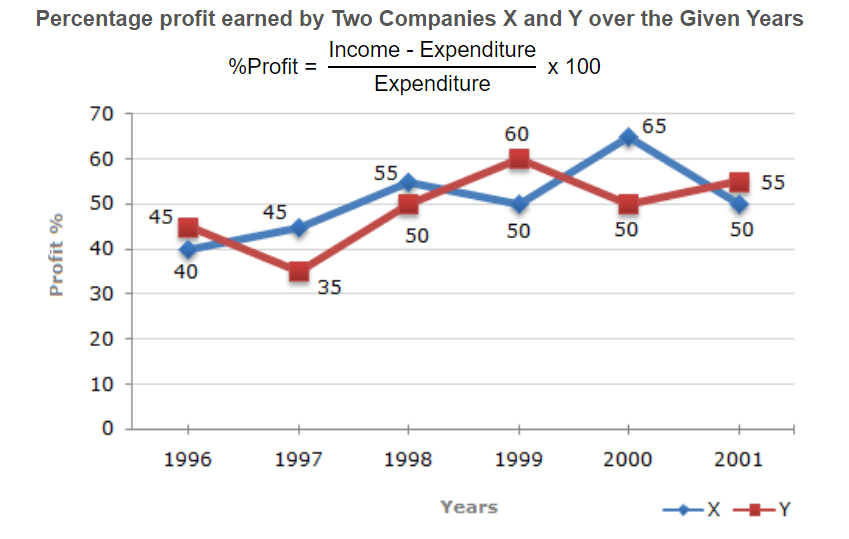# E-PolyLearning

 16. The following line graph gives the percent profit earned by two Companies X and Y during the period 1996 - 2001. The incomes of two Companies X and Y in 2000 were in the ratio of 3:4 respectively. What was the respective ratio of their expenditures in 2000 ?a. 7:22 b. 14:19 c. 15:22 d. 27:35

 17. The following line graph gives the percent profit earned by two Companies X and Y during the period 1996 - 2001. If the expenditure of Company Y in 1997 was Rs. 220 crores, what was its income in 1997 ? a. Rs. 312 crores b. Rs. 297 crores c. Rs. 283 crores d. Rs. 275 crores
 18. The following line graph gives the percent profit earned by two Companies X and Y during the period 1996 - 2001. If the expenditures of Company X and Y in 1996 were equal and the total income of the two Companies in 1996 was Rs. 342 crores, what was the total profit of the two Companies together in 1996 ? (Profit = Income - Expenditure)a. Rs. 240 crores b. Rs. 171 crores c. Rs. 120 crores d. Rs. 102 crores
 19. The following line graph gives the percent profit earned by two Companies X and Y during the period 1996 - 2001. The expenditure of Company X in the year 1998 was Rs. 200 crores and the income of company X in 1998 was the same as its expenditure in 2001. The income of Company X in 2001 was ?a. Rs. 465 crores b. Rs. 385 crores c. Rs. 335 crores d. Rs. 295 crores
 20. The following line graph gives the percent profit earned by two Companies X and Y during the period 1996 - 2001. If the incomes of two Comapanies were equal in 1999, then what was the ratio of expenditure of Company X to that of Company Y in 1999 ?a. 6:5 b. 5:6 c. 11:6 d. 16:15